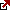# Forex Pip Calculator

Forex Pip Calculator is a tool traders use to calculate the value of a pip, which is a unit of measurement for the change in the value of a currency pair. A pip represents the smallest incremental change in the price of a currency pair, and its value depends on the size of the position, the currency pair being traded, and the current exchange rate.

To use the Forex Pip Calculator, you need to input the following parameters:

• Currency Pair: The first step is selecting the currency pair you want to trade. For example, if you are trading the EUR/USD pair, you would choose EUR/USD from the drop-down menu.
• Position Size: The second step is to enter the position size you want to trade. Position size is the amount of currency you are trading, typically measured in lots. For example, if you are trading one lot, the position size would be 100,000 units of the base currency.
• Pip Amount: The third step is to enter the number of pips by which you expect the currency pair’s price to move. This could be based on your analysis of market trends or your trading strategy.

Once you have entered these parameters, the Forex Pip Calculator will calculate the value of a pip, which is the change in the currency pair’s value for the given position size and pip amount. The value of a pip is expressed in the quote currency, the second currency in the currency pair.

For example, if you are trading one lot of the EUR/USD pair, and the value of a pip is \$10, then a 50 pip movement in the pair’s price would result in a profit or loss of \$500 (\$10 x 50 pips).

Overall, the Forex Pip Calculator is a valuable tool for traders to estimate the potential profit or loss of a trade based on the size of their position and the expected movement in the currency pair’s price. Using the calculator, traders can make more informed trading decisions and manage their risks more effectively.

## Forex Pip Value Formula

The formula for calculating the value of a pip using the Forex Pip Calculator is:

Pip Value = (Position Size * Pip Amount * Pip Value Quote Currency)/ Current Exchange Rate

Where:

Position Size is the size of the position, typically measured in lots.
Pip Amount is the number of pips by which you expect the currency pair’s price to move.
Pip Value Quote Currency is the value of one pip in the quote currency and the second currency in the currency pair.
The current Exchange Rate is the current price of the currency pair.
To use the Forex Pip Calculator with this formula, you would input the values for each of these variables, and the calculator will provide the result, which is the value of a pip in the quote currency.

For example, let’s say you want to trade one lot of the EUR/USD currency pair, and your trading strategy is based on a 50-pip movement in the pair’s price. The current exchange rate is 1.1800, and the value of one pip in the quote currency is \$10.

Using the formula, the calculation would be:

Pip Value = (1 * 50 * 10) / 1.1800 = \$423.73

This means that for a 50-pip movement in the price of the EUR/USD pair, you could profit or lose \$423.73 for a one lot position.

Overall, the Forex Pip Calculator uses this formula to calculate a pip’s value based on the position’s size, the expected movement in the currency pair’s price, and the current exchange rate. By using this tool, traders can more accurately estimate a trade’s potential risk and reward and make more informed trading decisions.

Latest posts by Fxigor (see all)

Fxigor

Igor has been a trader since 2007. Currently, Igor works for several prop trading companies. He is an expert in financial niche, long-term trading, and weekly technical levels. The primary field of Igor's research is the application of machine learning in algorithmic trading. Education: Computer Engineering and Ph.D. in machine learning. Igor regularly publishes trading-related videos on the Fxigor Youtube channel. To contact Igor write on: igor@forex.in.rs

Trade gold and silver. Visit the broker's page and start trading high liquidity spot metals - the most traded instruments in the world.Diversify your savings with a gold IRA.

Gold & silver portfolio-building assistance from product professionals.

If you want to trade stocks try: